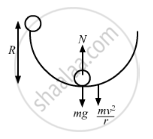Department of Pre-University Education, KarnatakaPUC Karnataka Science Class 11
Advertisement Remove all ads

# A Solid Sphere of Mass M is Released from Rest from the Rim of a Hemispherical Cup So that It Rolls Along the Surface. - Physics

Sum

A solid sphere of mass m is released from rest from the rim of a hemispherical cup so that it rolls along the surface. If the rim of the hemisphere is kept horizontal, find the normal force exerted by the cup on the ball when the ball reaches the bottom of the cup.

Advertisement Remove all ads

#### Solution

The radius of the solid sphere (r) is negligible w.r.t. the radius of the hemispherical cup (R).

Let the ball reaches the bottom of the cup with a velocity v and angular velocity ω.

On applying the law of conservation of energy, we get

$mgR = \frac{1}{2}I \omega^2 + \frac{1}{2}m v^2$$\Rightarrow mgR = \frac{1}{2} \times \frac{2}{5} m \nu^2 + \frac{1}{2} m \nu^2$

$\Rightarrow \frac{7}{10} m \nu^2 = mgR$

$\Rightarrow \nu^2 = \frac{10}{7}gR$

As shown in the free body diagram, we have

Total normal force on the ball $= mg + \frac{m \nu^2}{R}$

$= mg + \frac{m\left( \frac{10}{7}gR \right)}{R}$

$= mg + mg \left( \frac{10}{7} \right)$

$= \frac{17}{7}mg$

Concept: Momentum Conservation and Centre of Mass Motion
Is there an error in this question or solution?
Advertisement Remove all ads

#### APPEARS IN

HC Verma Class 11, 12 Concepts of Physics 1
Chapter 10 Rotational Mechanics
Q 77 | Page 200
Advertisement Remove all ads
Advertisement Remove all ads
Share
Notifications

View all notifications

Forgot password?